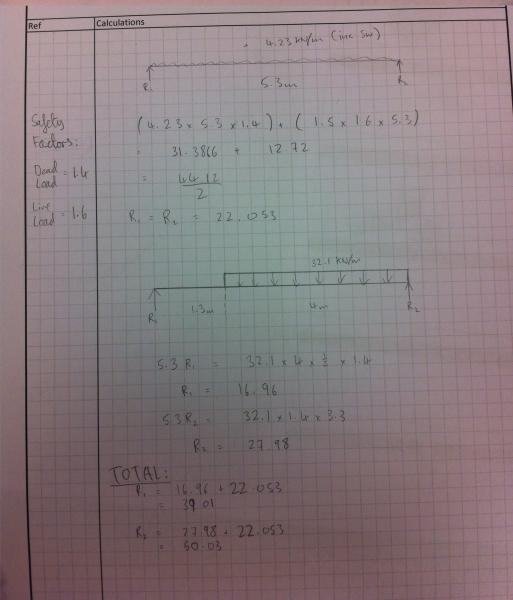# Shear and bending moment

Hi,

I am designing a beam to replace a load bearing wall.

I have produced 2 separate UDL diagrams which, one for floor load and one for wall load. I am trying to find shear force and bending moment as in one for the total of the two diagrams. I know how to do it if the loads are spread across the whole beam but when just part of it is loaded, thats where im a bit confused.

thanks

Here is what i have so far attached:## Answers and Replies

Your right, i was a little confused. I have understood what im doing now.

However im still not sure about finding the max deflection on a simply supported beam, when there are two UDL's.
For example, a 5m span beam has a udl of 2kN/m acting on 2m and 1kN/m acting on 3m. what is the formula to find max deflection?

Any advise is welcome.
Thank you

SteamKing
Staff Emeritus
Science Advisor
Homework Helper
You can use what is called superposition to find the combined deflection of a beam which has two different loadings.

In your case, you treat the beam as if it was loaded with only the 2 kN/m UDL acting on the 2m length and calculate the deflection at a series of different locations. Then, assume the beam is loaded with only the 1 kN/m UDL acting on the 3m length and calculate the deflection at the same locations as you did with the first UDL. The deflection of the beam with both UDLs applied will then be the sum of the deflections at each location for each loading.

thank you, i will give it a go :)